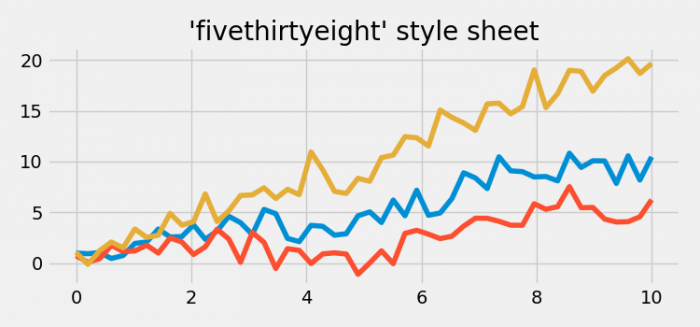# Plot curves in fivethirtyeight stylesheet in Matplotlib

To use fivethirtyeight stylesheet, we can take the following steps −

• Set the figure size and adjust the padding between and around the subplots.
• To use fivethirtyeight, we can use plt.style.use() method.
• Create x data points using numpy.
• Create a figure and a set of subplots using subplots() method.
• Plot three curves using plot() method.
• Set the title of the plot.
• To display the figure, use show() method.

## Example

import matplotlib.pyplot as plt
import numpy as np

plt.rcParams["figure.figsize"] = [7.50, 3.50]
plt.rcParams["figure.autolayout"] = True
plt.style.use('fivethirtyeight')

x = np.linspace(0, 10)
fig, ax = plt.subplots()

ax.plot(x, np.sin(x) + x + np.random.randn(50))
ax.plot(x, np.sin(x) + 0.5 * x + np.random.randn(50))
ax.plot(x, np.sin(x) + 2 * x + np.random.randn(50))
ax.set_title("'fivethirtyeight' style sheet")

plt.show()

## Output